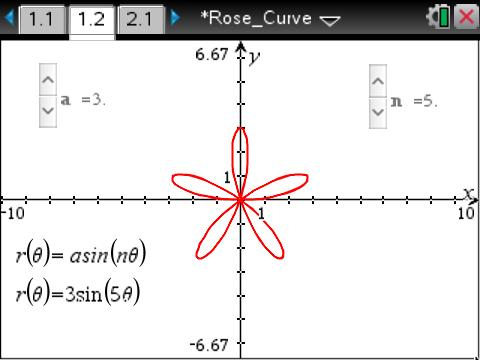# Activities

••• ##### Subject Area

• Math: Precalculus: Applications of Trigonometry

• ##### Author9-12

45 Minutes

• ##### Device
•TI-Nspire™ CX/CX II
•TI-Nspire™ CX CAS/CX II CAS
• TI-Nspire™ Navigator™
• ##### Software

TI-Nspire™ CX
TI-Nspire™ CX CAS

3.6

• ##### Report an Issue

Rose Curve#### Activity Overview

This lesson involves clicking on sliders to observe the effect of changing the values of a and n in the equation r = asin().

#### Objectives

• Students will understand the role of the values of a and n in the equation r = asin(nθ).
• Students will be able to predict the number of petals and their length by examining the polar equation.
• Student will understand the relationship between the equation of a rose curve and the equation of a sinusoidal function.

#### Vocabulary

• amplitude
• frequency
• rose curve
• sinusoidal function